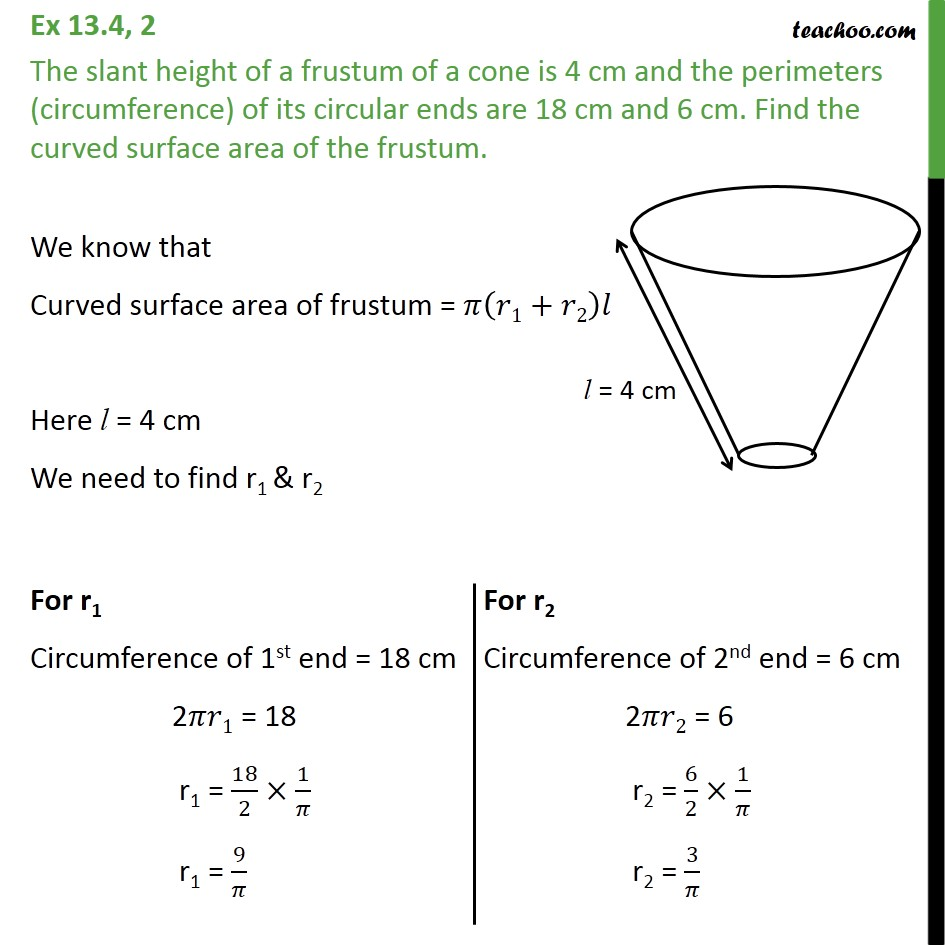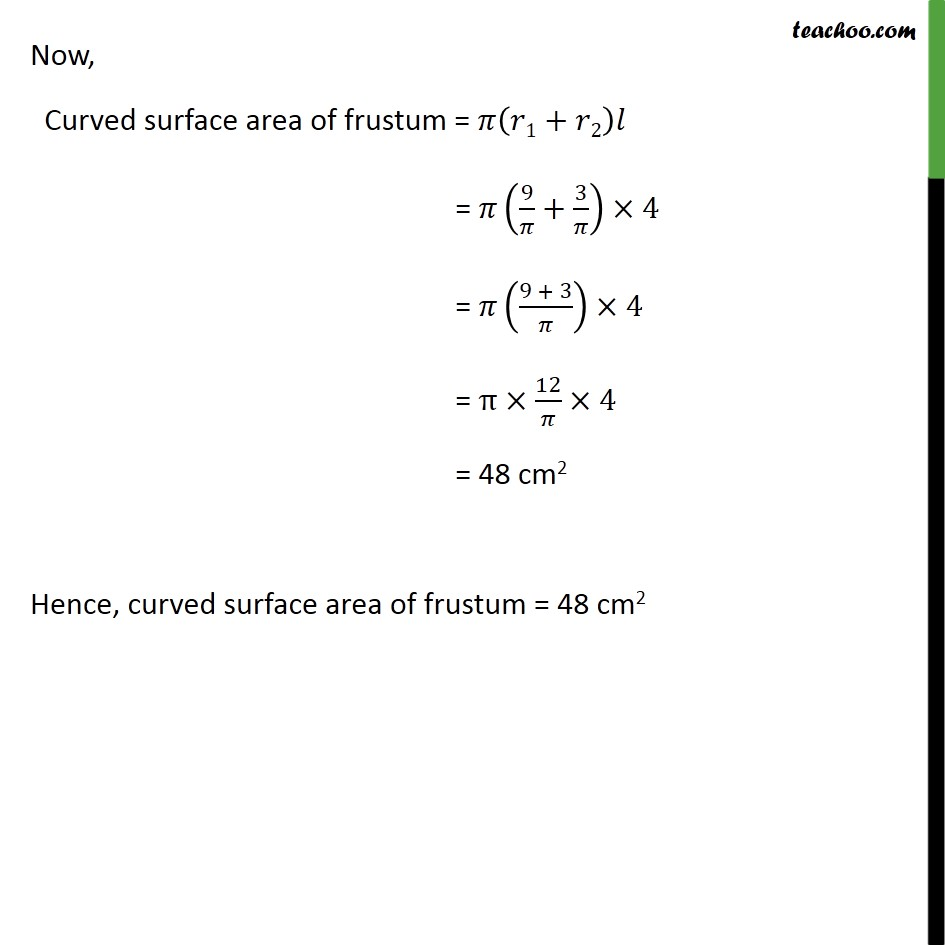Frustum - Area and Volume

Chapter 12 Class 10 Surface Areas and Volumes
Serial order wiseLearn in your speed, with individual attention - Teachoo Maths 1-on-1 Class

### Transcript

Question 2 The slant height of a frustum of a cone is 4 cm and the perimeters (circumference) of its circular ends are 18 cm and 6 cm. Find the curved surface area of the frustum. We know that Curved surface area of frustum = ( 1+ 2) Here l = 4 cm We need to find r1 & r2 Now, Curved surface area of frustum = ( 1+ 2) = (9/ +3/ ) 4 = ((9 + 3)/ ) 4 = 12/ 4 = 48 cm2 Hence, curved surface area of frustum = 48 cm2